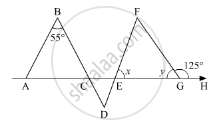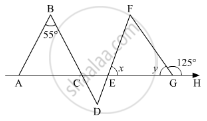Advertisement Remove all ads

# In the Given Figure, If Ab || De And Bd || Fg Such that ∠Fgh = 125° and ∠B = 55°, Find X And Y. - Mathematics

Answer in Brief

In the given figure, if AB || DE and BD || FG such that ∠FGH = 125° and ∠B = 55°, find x and y.Advertisement Remove all ads

#### Solution

In the given figure,,,and  AB ||DE ,BD || FG , ∠FGH =125° and ∠B = 55°

We need to find the value of and yHere, as AB || DE and BD is the transversal, so according to the property, “alternate interior angles are equal”, we get

∠D = ∠B

∠D = 55°            ............. (1)

Similarly, as BD || FG and DF is the transversal

∠D = ∠F

∠F = 55° (Using 1)

Further, EGH is a straight line. So, using the property, angles forming a linear pair are supplementary

∠FGE + ∠FGH = 180°

y + 125° = 180°

y = 180° - 125°

y = 55°

Also, using the property, “an exterior angle of a triangle is equal to the sum of the two opposite interior angles”, we get,

In  ΔEFG with ∠ FGH as its exterior angle

ext.  ∠FGH = ∠F + ∠E

125° = 55° + x

x = 125° - 55°

x = 70°

Thus, x = 70° and y = 55°

Is there an error in this question or solution?
Advertisement Remove all ads

#### APPEARS IN

RD Sharma Mathematics for Class 9
Chapter 11 Triangle and its Angles
Q 8 | Page 24
Advertisement Remove all ads

#### Video TutorialsVIEW ALL 

Advertisement Remove all ads
Share
Notifications

View all notifications

Forgot password?
Course